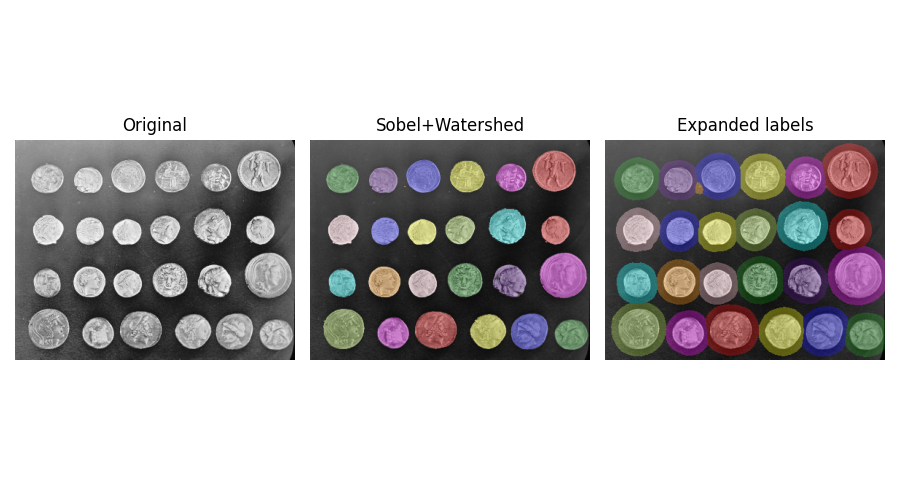# Expand segmentation labels without overlap#

Given several connected components represented by a label image, these connected components can be expanded into background regions using `skimage.segmentation.expand_labels()`. In contrast to `skimage.morphology.dilation()` this method will not let connected components expand into neighboring connected components with lower label number.```import matplotlib.pyplot as plt
import numpy as np
from skimage import data
from skimage.color import label2rgb
from skimage.filters import sobel
from skimage.measure import label
from skimage.segmentation import expand_labels, watershed

coins = data.coins()

# Make segmentation using edge-detection and watershed.
edges = sobel(coins)

# Identify some background and foreground pixels from the intensity values.
# These pixels are used as seeds for watershed.
markers = np.zeros_like(coins)
foreground, background = 1, 2
markers[coins < 30.0] = background
markers[coins > 150.0] = foreground

ws = watershed(edges, markers)
seg1 = label(ws == foreground)

expanded = expand_labels(seg1, distance=10)

# Show the segmentations.
fig, axes = plt.subplots(
nrows=1,
ncols=3,
figsize=(9, 5),
sharex=True,
sharey=True,
)

axes.imshow(coins, cmap="Greys_r")
axes.set_title("Original")

color1 = label2rgb(seg1, image=coins, bg_label=0)
axes.imshow(color1)
axes.set_title("Sobel+Watershed")

color2 = label2rgb(expanded, image=coins, bg_label=0)
axes.imshow(color2)
axes.set_title("Expanded labels")

for a in axes:
a.axis("off")
fig.tight_layout()
plt.show()
```

Total running time of the script: ( 0 minutes 0.792 seconds)

Gallery generated by Sphinx-Gallery